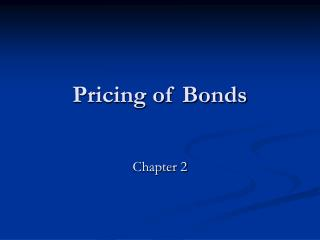DownloadDownload PresentationPricing of Bonds

# Pricing of Bonds

Télécharger la présentation## Pricing of Bonds

- - - - - - - - - - - - - - - - - - - - - - - - - - - E N D - - - - - - - - - - - - - - - - - - - - - - - - - - -
##### Presentation Transcript

1. Pricing of Bonds Chapter 2

2. Time of Value • Future Value where: n = number of periods Pn = future value n periods from now (in dollars) Po = original principal (in dollars) r = interest rate per period (in decimal form) • Future Value of on Ordinary Annuity

3. Time Value of Money • Present Value • Present Value of a Series of Future Values

4. Time Value of Money • Present Value of an Ordinary Annuity

5. Bond Pricing • price = PV of all future cash flows • to find price, you need • expected CFs • coupon payments • par value • yield

6. Bond Pricing • price – yield relationship • inverse because as yield falls, PV of CFs increases and price of bond increases • price-yield curve is convex for noncallable bonds • discount / premium to par when coupon rate < / > required yield • market price changes when • yield changes due to credit quality of issuer • change in price of bond selling at discount/premium because getting closer to maturity • change in yield required by market for bonds of similar risk

7. Bond Pricing • floater – security that has coupon rate that changes when market rates change • coupon = reference rate plus spread • reset periodically • cap / floor • inverse floater • created using a fixed rate bond (collateral) • create floater and inverse floater so that • total coupon interest paid to two bonds in each period is less than or equal to collateral’s coupon interest in each period • total par value of two bonds is less than or equal to collateral’s total par value • ie., two securities created so that CFs from collateral can satisfy obligation of floater and inverse floater

8. Prices Converted into a Dollar Price